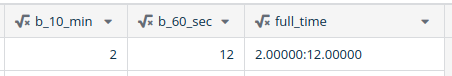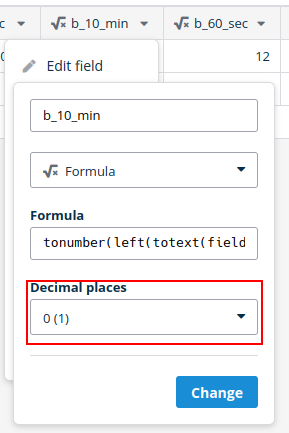# Concat not functioning as expected with numbers from Formula fields

I’m trying to concatenate `b_10_min` and `b_60_sec` into a time format like so `2:12`. In fact 2:12 was the result I was expecting. As you can see (below) the result I’m getting is, off… lolHere is the logic behind the `full_time` fields:
`concat(totext(field('b_10_min')), ':', totext(multiply(field('b_10_sec'), 60)))`

I’m surprised to see the trailing zeros in the `full_time` field because the fields that the numbers are coming from (`b_10_min` and `b_60_sec`) are set to no decimal places:Logic behind `b_10_min`:
`tonumber(left(totext(field('b_10_pace')), minus(field('b_10_pace'), length(totext(field('b_10_pace'))))))`

Logic behind `b_60_sec`:
`multiply(tonumber(right(totext(field('b_10_pace')), minus(length(totext(field('b_10_pace'))), add(search(totext(field('b_10_pace')), '.'), -1)))), 60)`

Hello @baserow.rvor6! We’ll check this case, and sorry for the inconvenience this causes!

There’s a few things going on here to understand why these formulas are generating results with 5DP for you.

1. When you reference a formula from another formula in the same table, internally we literally substitute the referenced formula into the referencing formula.

For example if you have two formulas:

• Formula A is `10*20/30`
• Formula B is `field('Formula A') * 30`
When calculating the results for Formula B Baserow will substitute in Formula A and calculate the following formula: `(10*20/30) * 30`
1. When you set the decimal places in the field edit box for a formula field, this doesn’t affect the above substitution in anyway. So changing the DP for Formula A above will have no affect on the number of DP in Formula B.

2. So why are the numbers in full_time ending up with 5 DP? Well I believe this is because you ultimately are referencing `time_min` and `time_sec`, whose formula’s seem to be calculating a result with 5 decimal places. Due to the formula substitution going on `full_time` will be calculated as one big formula and inherit the “auto calculated” decimal places coming out of the `time_min` and `time_sec` formulas.

## The solution?

Could you provide the formulas for `time_min`, `time_sec` and `b_10_pace`? There is hopefully some way of modifying them to generate the desired number of DP. In the next version of Baserow we will be releasing `round` and `trunc` functions which will let you explicitly set the desired number of DP in a formula.

## Alternatives

When designing how formulas behaved we weren’t 100% sure what to do in this exact situation you encountered. That is:

1. I create some number formula, I set the DP to be 5 but the underlying formula calculates a numeric result that uses the max level of 10 DP of precision like `10/3`.
2. When another formula references this formula should we treat the user setting the DP as an implicit `trunc` function call? Or should we instead just substitute and provide the referencing formula as much precision as possible.

We went with “substitute exactly and provide referencing formulas as much precision” reasoning that the decimal places you choose in the field options are just for display purposes. So for example you might want to look at some intermediate formula result but only see 2 DP but pass the full precision down to any referencing formulas.

We could alternatively treat the selection of decimal places at a field level for a formulas as an implicit “trunc” function call wrapping that formula, and so that would be passed down to all other referencing formulas. However with the inclusion of the `trunc`/`round` functions it seems to me that doing this implicitly perhaps is less flexible. But we’re completely open to other ideas here